# Samacheer Kalvi 12th Maths Solutions Chapter 7 Applications of Differential Calculus Ex 7.9

## Tamilnadu Samacheer Kalvi 12th Maths Solutions Chapter 7 Applications of Differential Calculus Ex 7.9

Question 1.
Find the asymptotes of the following curves: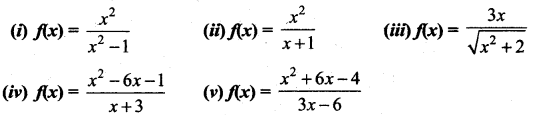Solution: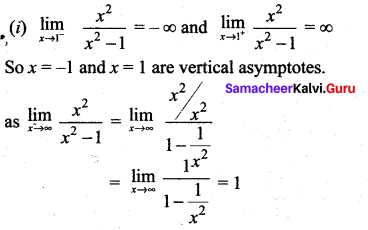y = 1 is a horizontal asymptote
So the asymptotes are x = -1, x = +1, y = 1(ii) Since the numerator is of higher degree than the denominator we have a slant asymptote to find that asymptote we have to divide the numerator by the denominator So the slant asymptote is y = x – 1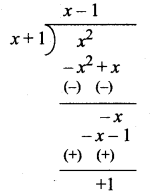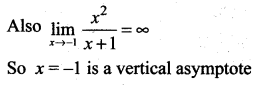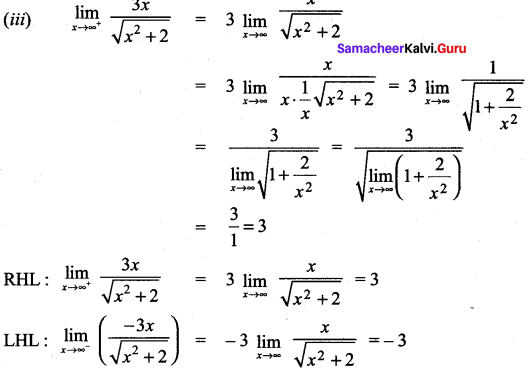∴ y = 3 and y = -3 are the horizontal asymptotes and there is no slant asymptote
(iv) Since the numerator is of highest degree than the denominator. We have a slant asymptote to find it we have to divide numerator by the denominator.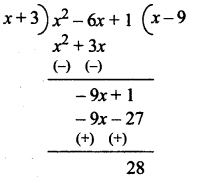So the equation of asymptotes is y = x – 9 and x = -3
(v) Since the numerator is of highest degree than the denominator. We have a slant asymptote to find it we have to divide the numerator by the denominator.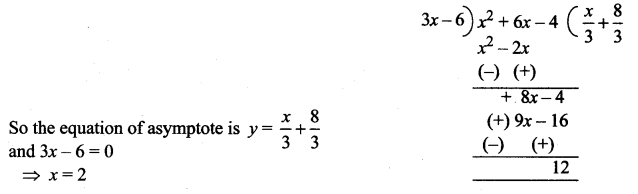Question 2.
Sketch the graphs of the following functions:Solution: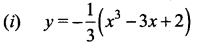Factorizing we get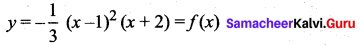• The domain and the range of the given function f(x) are the entire real line.
• Putting y = 0 we get x = 1, 1, -2. Hence the x intercepts are (1, 0) and (-2, 0) and by putting x = 0 we get y = $$-\frac{2}{3}$$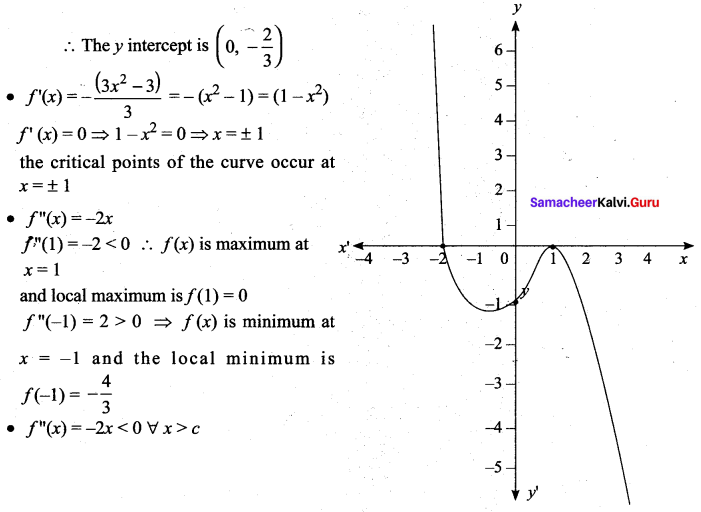∴ The function is concave upward in the negative real line.
• Since f”(x) = 0 at x = 0 and f”(x) changes its sign when passing through x = 0, x = 0 is a point of inflection is $$\left(0,-\frac{2}{3}\right)$$
• The curve has no asymptotes.

(ii)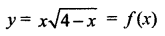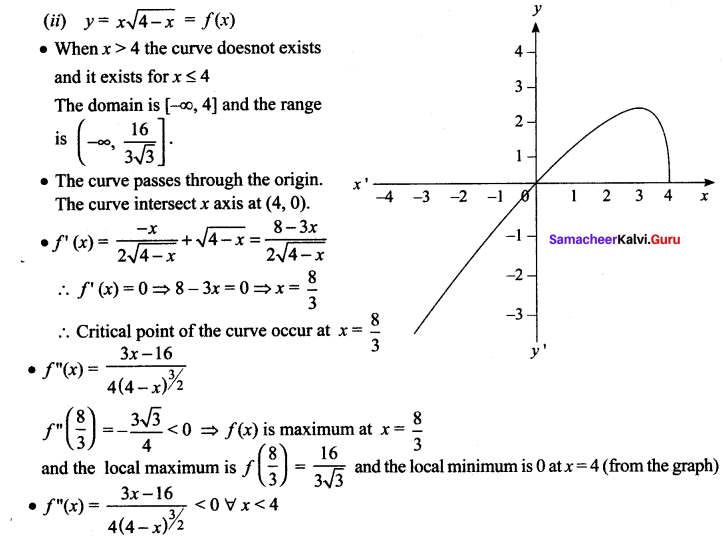The curve concave downward in the negative real line
• No point of inflection exists.
• as x ➝ ∞, y = ± ∞ and so the curve does not have any asymptotes

(iii)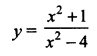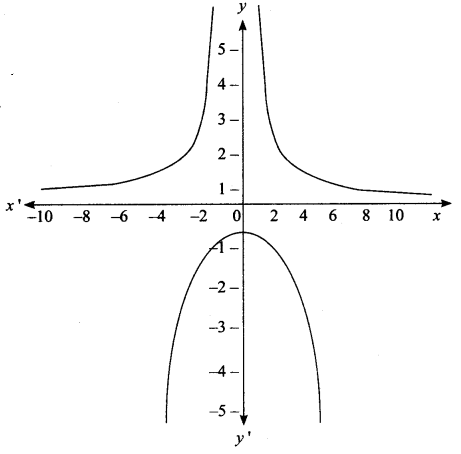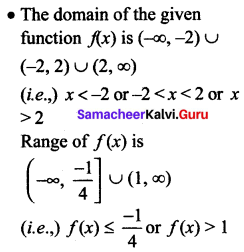• Putting y = 0. x is unreal hence there is no ‘x’ intercept. By putting x = 0 we get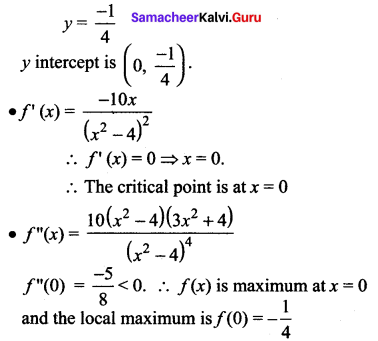• No points of reflection
• When x = ± 2, y = ∞, Vertical asymptotes are x = 2 and x = -2 and horizontal asymptote is y = 1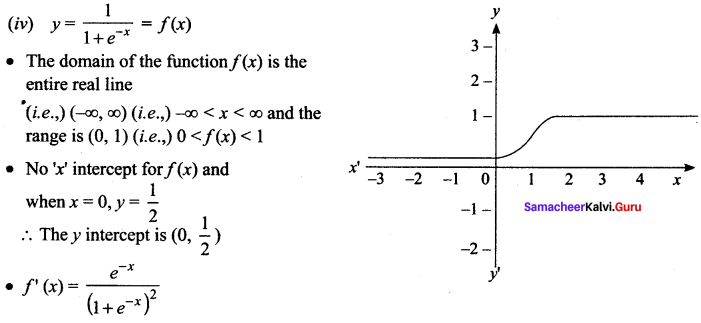f'(x) = 0 ⇒ e-x = 0 which is not possible hence there is no extremum.
• No vertical asymptote for the curve and the horizontal asymptotes are y = 1 and y = 0(v)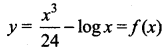• The curve exists only for positive values of (x > 0)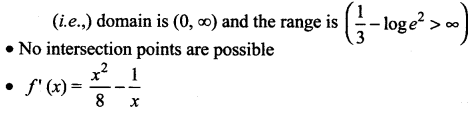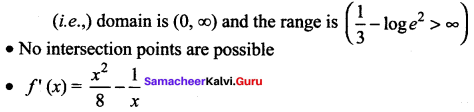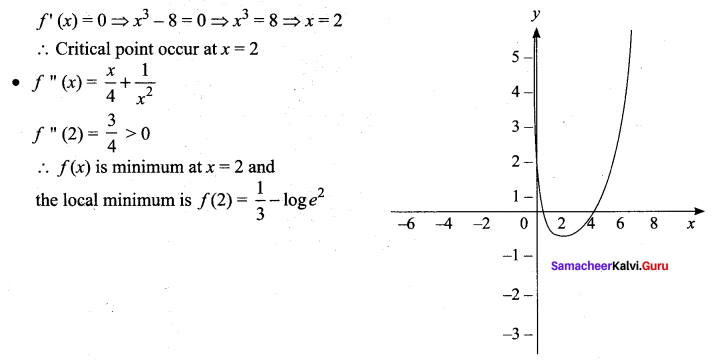• No point of inflection.
• No horizontal asymptote is possible.
But the vertical asymptote is x = 0(y axis).